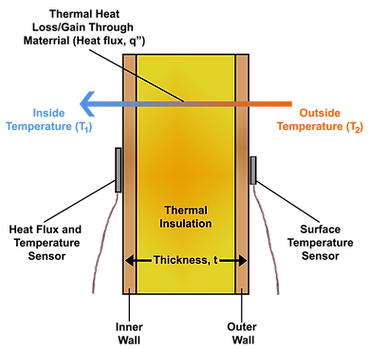top of page###### Measuring Thermal Insulation R-value

Note: Using heat flux sensors is the only way to perform in-situ measurements of R-value of building envelope components and has been standardized in ASTM C1046 and ASTM C1155.

Heat flux sensors are the only sensors that can be used to take in-situ measurements of material thermal resistance, commonly referred to as R-Value. Other methods are either destructive to the material or are unable to a provided quantitative R-value numbers. Even using original manufacturer’s specifications for a material does not account for factors that affect the thermal performance such as degradation over time, humidity or physical damage.

The PHFS product line of heat flux sensor utilize a differential-temperature thermopile design to measure the movement of thermal energy per unit area, or heat flux, through the sensor surface. Each PHFS sensor includes an integrated thermocouple that can be used for sensor temperature measurements. For measuring relatively low heat fluxes usually seen in R-value measurement applications, FluxTeq recommends using our PHFS-09e heat flux sensors that have excellent sensitivity.

Theory Behind R-value Measurements

The R-value of thermal insulation can be determined through a few simple measurements that are made possible using the PHFS heat flux sensors. A diagram of heat transfer through a building wall is shown below.The underlying equation for determining the R-value of a material originated from Fourier’s law of conduction that assumes steady-state one-dimensional heat transfer through the material.

Where q” is the one-dimensional heat flux through the material, k is the thermal conductivity, and t is the thickness.  From the above equation it can be seen that there exists three unknown variables, heat flux (q”), T2(outside), and T1(inside), that are required in order to determine the material’s thermal resistance R-value.  All of these values can be obtained using measurement from 2 PHFS heat flux sensors that are positioned on both the inside and outside surfaces of the material being tested.  Note: The measurement of R-value can be taken using a single PHFS heat flux sensor located on one side of the material surface with just a surface temperature sensor located on the opposite wall but FluxTeq recommends using two to double-check that the heat flux is approximately one-dimensional/equal through both measurement surfaces.

The measurements should be taken over an extended period of time and then analyzed to determine the overall effective R-value of the material.

A full description of the process and tips for taking in-situ measurements of thermal resistance R-value can be found in PDF document attached to this page.

bottom of page